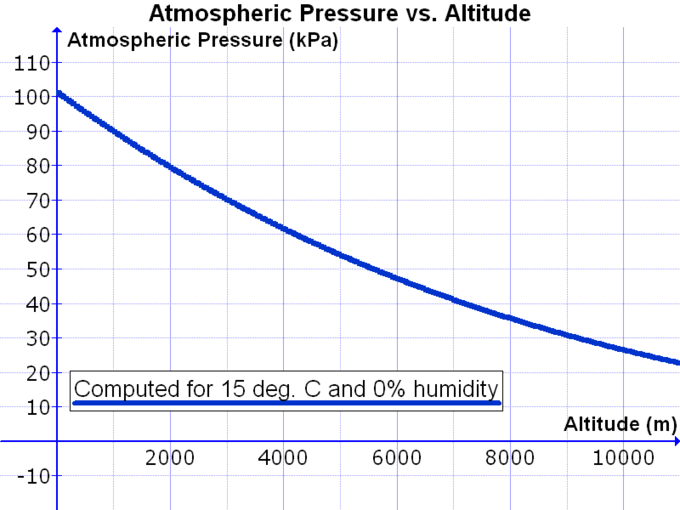# 39.5: Gas Exchange across Respiratory Surfaces - Gas Pressure and Respiration

•• Boundless
• Boundless
$$\newcommand{\vecs}{\overset { \rightharpoonup} {\mathbf{#1}} }$$ $$\newcommand{\vecd}{\overset{-\!-\!\rightharpoonup}{\vphantom{a}\smash {#1}}}$$$$\newcommand{\id}{\mathrm{id}}$$ $$\newcommand{\Span}{\mathrm{span}}$$ $$\newcommand{\kernel}{\mathrm{null}\,}$$ $$\newcommand{\range}{\mathrm{range}\,}$$ $$\newcommand{\RealPart}{\mathrm{Re}}$$ $$\newcommand{\ImaginaryPart}{\mathrm{Im}}$$ $$\newcommand{\Argument}{\mathrm{Arg}}$$ $$\newcommand{\norm}{\| #1 \|}$$ $$\newcommand{\inner}{\langle #1, #2 \rangle}$$ $$\newcommand{\Span}{\mathrm{span}}$$ $$\newcommand{\id}{\mathrm{id}}$$ $$\newcommand{\Span}{\mathrm{span}}$$ $$\newcommand{\kernel}{\mathrm{null}\,}$$ $$\newcommand{\range}{\mathrm{range}\,}$$ $$\newcommand{\RealPart}{\mathrm{Re}}$$ $$\newcommand{\ImaginaryPart}{\mathrm{Im}}$$ $$\newcommand{\Argument}{\mathrm{Arg}}$$ $$\newcommand{\norm}{\| #1 \|}$$ $$\newcommand{\inner}{\langle #1, #2 \rangle}$$ $$\newcommand{\Span}{\mathrm{span}}$$

Gas pressures in the atmosphere and body determine gas exchange: both O2 and CO2 will flow from areas of high to low pressure.

##### Learning Objectives
• Describe how gas pressure influences the flow of gases during respiration

## Key Points

• Atmospheric pressure is the sum of all the partial pressures of the gases in the atmosphere, including oxygen, carbon dioxide, nitrogen, and water vapor.
• In the atmosphere, the partial pressure of oxygen is much greater than the partial pressure of carbon dioxide.
• The partial pressure of oxygen in the atmosphere is much greater in comparison to the lungs, creating a pressure gradient; this allows oxygen to flow from the atmosphere into the lungs during inhalation.

## Key Terms

• atmospheric pressure: the pressure caused by the weight of the atmosphere above an area
• partial pressure: the pressure one component of a mixture of gases would contribute to the total pressure

## Gas Pressure and Respiration

The respiratory process can be better understood by examining the properties of gases. Gases move freely with their movement resulting in the constant hitting of particles against vessel walls. This collision between gas particles and vessel walls produces gas pressure.

Air is a mixture of gases: primarily nitrogen (N2; 78.6 percent), oxygen (O2; 20.9 percent), water vapor (H2O; 0.5 percent), and carbon dioxide (CO2; 0.04 percent). Each gas component of that mixture exerts a pressure. The pressure for an individual gas in the mixture is the partial pressure of that gas. Approximately 21 percent of atmospheric gas is oxygen. Carbon dioxide, however, is found in relatively small amounts (0.04 percent); therefore, the partial pressure for oxygen is much greater than that of carbon dioxide. The partial pressure of any gas can be calculated by: P = (Patm) (percent content in mixture).

Patm, the atmospheric pressure, is the sum of all of the partial pressures of the atmospheric gases added together: Patm = PN2 + PO2 + PH2O + PCO2= 760 mm Hg. The pressure of the atmosphere at sea level is 760 mm Hg. Therefore, the partial pressure of oxygen is: PO2 = (760 mm Hg) (0.21) = 160 mm Hg, while for carbon dioxide: PCO2 = (760 mm Hg) (0.0004) = 0.3 mm Hg. At high altitudes, Patmdecreases, but concentration does not change; the partial pressure decrease is due to the reduction in Patm .Figure $$\PageIndex{1}$$: Atmospheric pressure vs altitude: At high altitudes, there is a decrease in Patm, causing the partial pressures to decrease as well.

When the air mixture reaches the lung, it has been warmed and humidified within the nasal cavity upon inhalation. The pressure of the water vapor in the lung does not change the pressure of the air, but it must be included in the partial pressure equation. For this calculation, the water pressure (47 mm Hg) is subtracted from the atmospheric pressure: 760 mm Hg 47 mm Hg = 713 mm Hg, and the partial pressure of oxygen is: (760 mm Hg 47 mm Hg) 0.21 = 150 mm Hg.

These pressures determine the gas exchange, or the flow of gas, in the system. Oxygen and carbon dioxide will flow according to their pressure gradient from high to low. Therefore, understanding the partial pressure of each gas will aid in understanding how gases move in the respiratory system.

39.5: Gas Exchange across Respiratory Surfaces - Gas Pressure and Respiration is shared under a CC BY-SA 4.0 license and was authored, remixed, and/or curated by Boundless.